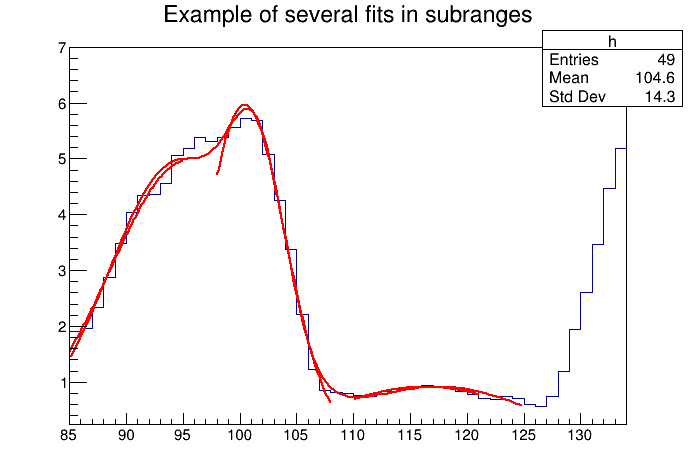# Multifit¶

Example showing how to fit in a sub-range of an histogram An histogram is created and filled with the bin contents and errors defined in the table below. 3 gaussians are fitted in sub-ranges of this histogram. A new function (a sum of 3 gaussians) is fitted on another subrange Note that when fitting simple functions, such as gaussians, the initial values of parameters are automatically computed by ROOT. In the more complicated case of the sum of 3 gaussians, the initial values of parameters must be given. In this particular case, the initial values are taken from the result of the individual fits.

Author: Wim Lavrijsen
This notebook tutorial was automatically generated with ROOTBOOK-izer from the macro found in the ROOT repository on Thursday, June 04, 2020 at 02:46 AM.

In :
from ROOT import TH1F, TF1
from ROOT import gROOT
from array import array

x = ( 1.913521, 1.953769, 2.347435, 2.883654, 3.493567,
4.047560, 4.337210, 4.364347, 4.563004, 5.054247,
5.194183, 5.380521, 5.303213, 5.384578, 5.563983,
5.728500, 5.685752, 5.080029, 4.251809, 3.372246,
2.207432, 1.227541, 0.8597788,0.8220503,0.8046592,
0.7684097,0.7469761,0.8019787,0.8362375,0.8744895,
0.9143721,0.9462768,0.9285364,0.8954604,0.8410891,
0.7853871,0.7100883,0.6938808,0.7363682,0.7032954,
0.6029015,0.5600163,0.7477068,1.188785, 1.938228,
2.602717, 3.472962, 4.465014, 5.177035 )

np = len(x)
h = TH1F( 'h', 'Example of several fits in subranges', np, 85, 134 )
h.SetMaximum( 7 )

for i in range(np):
h.SetBinContent( i+1, x[i] )

par = array( 'd', 9*[0.] )
g1 = TF1( 'g1', 'gaus',  85,  95 )
g2 = TF1( 'g2', 'gaus',  98, 108 )
g3 = TF1( 'g3', 'gaus', 110, 121 )

total = TF1( 'total', 'gaus(0)+gaus(3)+gaus(6)', 85, 125 )
total.SetLineColor( 2 )
h.Fit( g1, 'R' )
h.Fit( g2, 'R+' )
h.Fit( g3, 'R+' )

par1 = g1.GetParameters()
par2 = g2.GetParameters()
par3 = g3.GetParameters()

par, par, par = par1, par1, par1
par, par, par = par2, par2, par2
par, par, par = par3, par3, par3

total.SetParameters( par )
h.Fit( total, 'R+' )

Welcome to JupyROOT 6.23/01

Out:
<cppyy.gbl.TFitResultPtr object at 0x55814e8c6eb0>

Draw all canvases

In :
from ROOT import gROOT
gROOT.GetListOfCanvases().Draw()